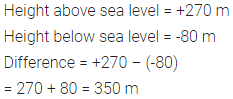ML Aggarwal Class 6 Solutions Chapter 3 Integers Check Your Progress for ICSE Understanding Mathematics acts as the best resource during your learning and helps you score well in your exams.

## ML Aggarwal Class 6 Solutions for ICSE Maths Chapter 3 Integers Check Your Progress

Question 1.
Use the appropriate symbol < or > to fill in the following blanks:
(i) (-3 + ……… (-6) (-3) – (-6)
(ii) (-21) – (-10) ……. (-31)+ (-11)
(iii) 45 – (-11) ……….. (57) + (-4)
(iv) (-25) – (-42) …………. (-42) – (-25)
Solution:Question 2.
Find the value of:
(i) 12 + ( -3) + 5 – (-2)
(ii) 39 – 35 + 7-(-4) + 21
(iii) -15- (-2) – 71 – 8 + 6
Solution: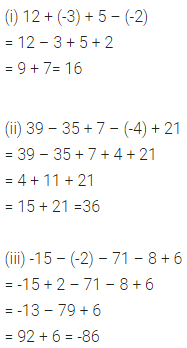Question 3.
Evaluate:
(i) |-13| – |-15|
(ii) |35 – 41| – |7-(-2)|
Solution: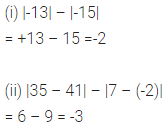Question 4.
Arrange the following integers in ascending order:
-39, 35, -102, 0, -51, -5, -6, 7
Solution:Question 5.
Find the successor and the predecessor of -199.
Solution: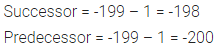Question 6.
Subtract the sum of -235 and 137 from -152.
Solution: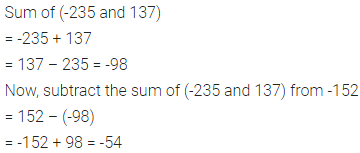Question 7.
What must be added to -176 to get -95?
Solution:Question 8.
What is the difference in height between a point 270 m above sea level and 80 m below sea level?
Solution: